# SUGMs¶

• Nature/people form Sj subnetworks of type j each independently with probability pj
• May intersect and overlap
• Weobserveresultingnetwork, infer the $p_j’s$

## Estimation - Two Approaches¶

• Sparse graphs: rare incidentals, Direct estimation is valid / consistent
• Algorithm: corrects for small n, and provides estimates for non-sparse (see CJ paper)

## Sparseness¶

• Incidentals generated by combinations of other subgraphs
• Sparsity definition relates rates of all subgraphs to each other (none grow too quickly)
• Intutive example: links and triangles
• $p_L = O(n^{-1/2})$, $p_T = O(n^{-3/2})$
• Typical node involved in less than $n^{1/2}$ links, $n^{1/2}$ triangles

# Links Form: Incidental Triangle¶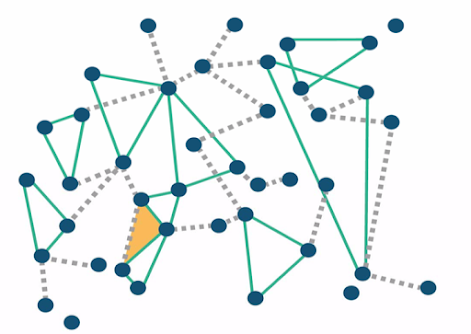# Estimation¶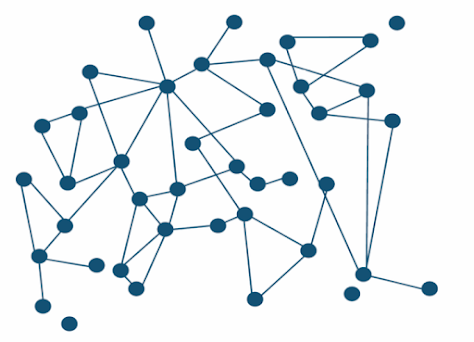# Estimation: Triangles¶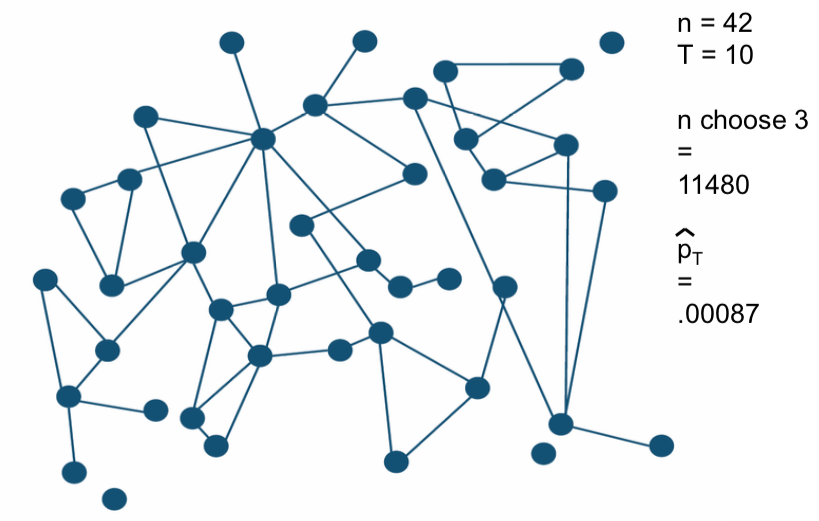number of nodes = 42

number of triangles = 10

n choose 3 = 424140 / 321 = 11480

$\hat p_t = 10 / 11480 = .00087$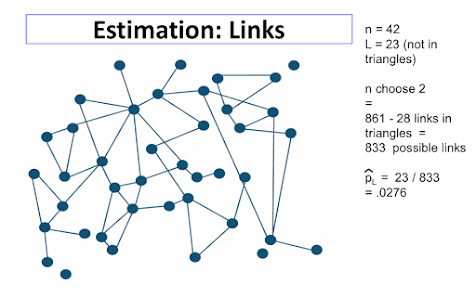## Theorem: Consistency and Distribution¶

Consider a sequence of sparse SUGMs

$S$: how many did you actually observe

$\bar s$: how many could there have been

The empirical frequency $\hat p^n_j = S^n_j / \bar S^n_j$ is (ratio) consistent:

$\hat p^n_j / p^n_j -> 1$ and $D^{1/2}(\hat p^n - p^n) -> N(0, 1)$

## Need for SERGMs/SUGMs:¶

• Examine data from 75 Indian villages from BCDJ '13
• How well do the model-recreated networks match real networks on non-modeled characteristics
• Estimate SUGM based on covariates, allowing for triangle counts
• Estimate standard link-based (block) model based on covariates
• Does SUGM do better than block model at recreating networks?
• Block model
• prob of a link of both of the same category or similar to each other
• prob of link if different
• SUGM add in
• prob of triangle if all similar
• prob of triangle if some different

block model is a special case of a SUGM where we just look at links

• Step 1: Estimate models
• Block model, estimate $p_{LinkSame}$ $p_{LinkDiff}$
• SUGM, estimate $p_{LinkSame}$ $p_{LinkDiff}$, $p_{TriadSame}$ $p_{TriadDiff}$
• Step 2: randomly generate networks
• Block model - randomly generate links
• SUGM - randomly generate links, triangles...
• Step 3: After generate networks, we can see whether or not these networks recreate the actual, original observations.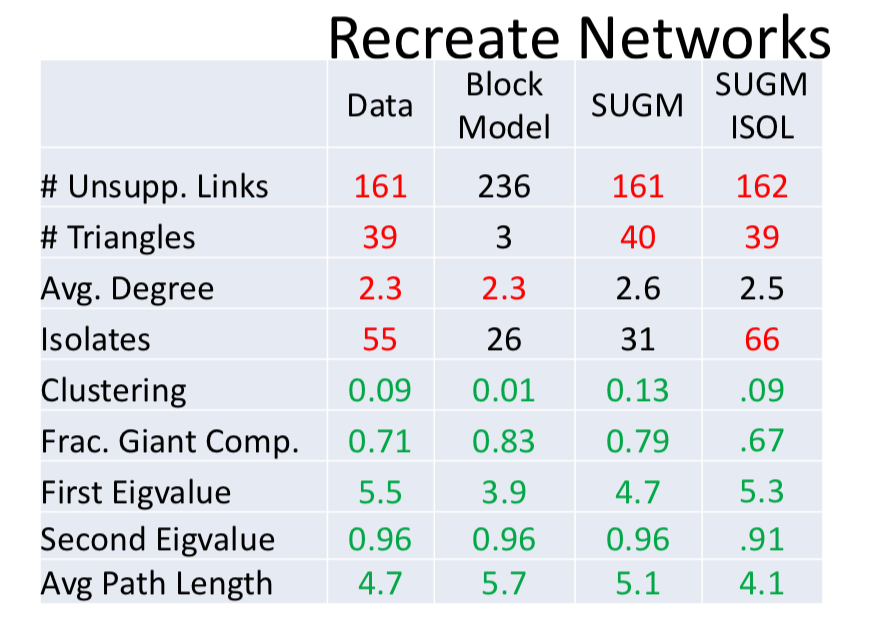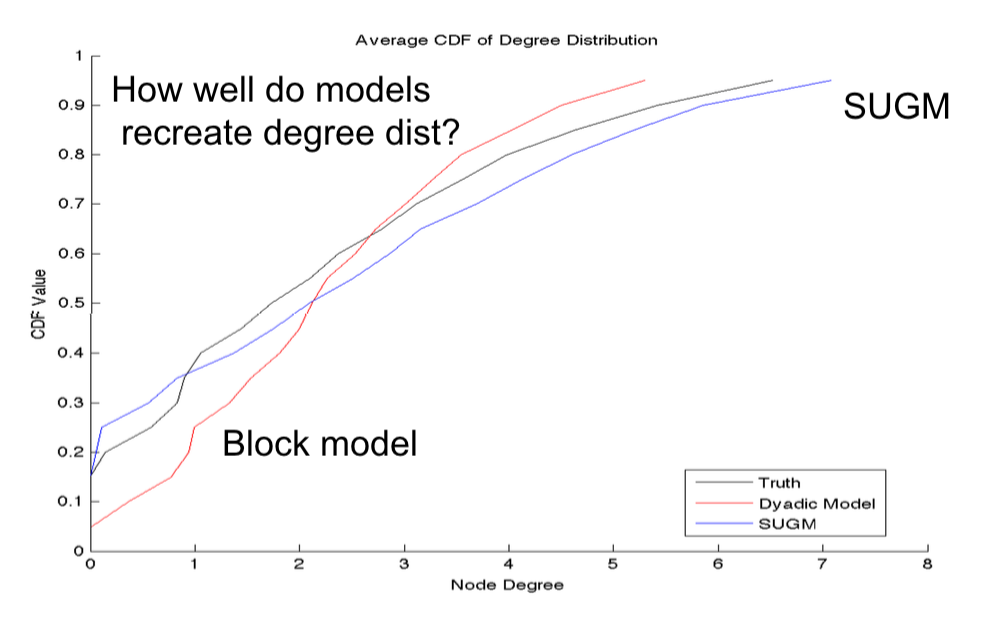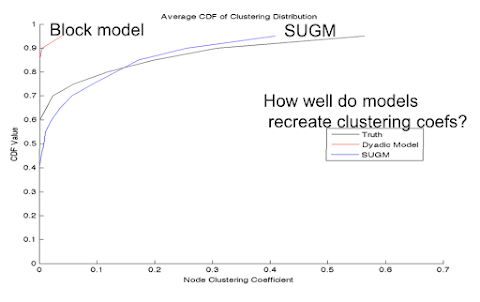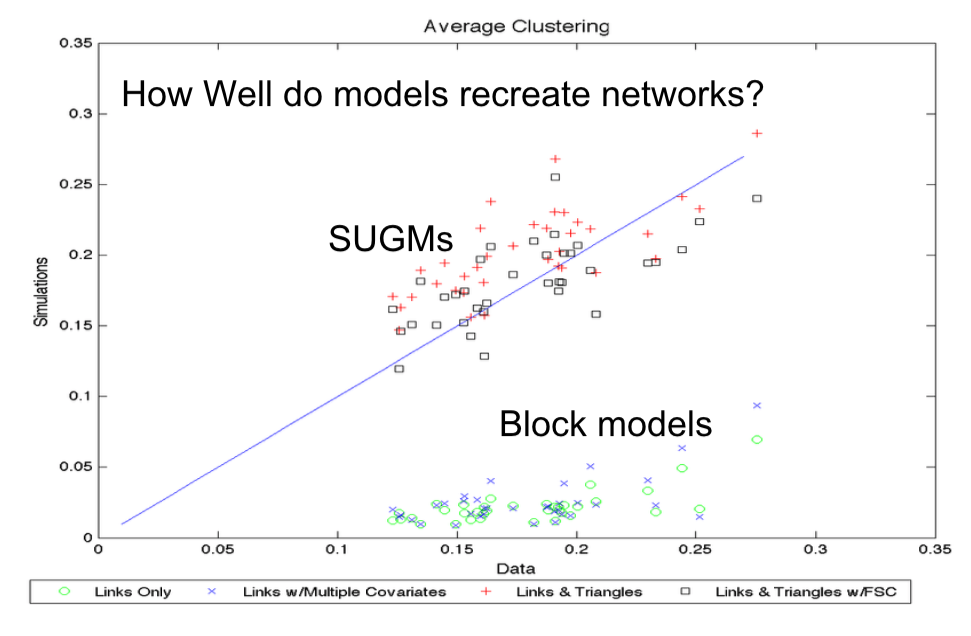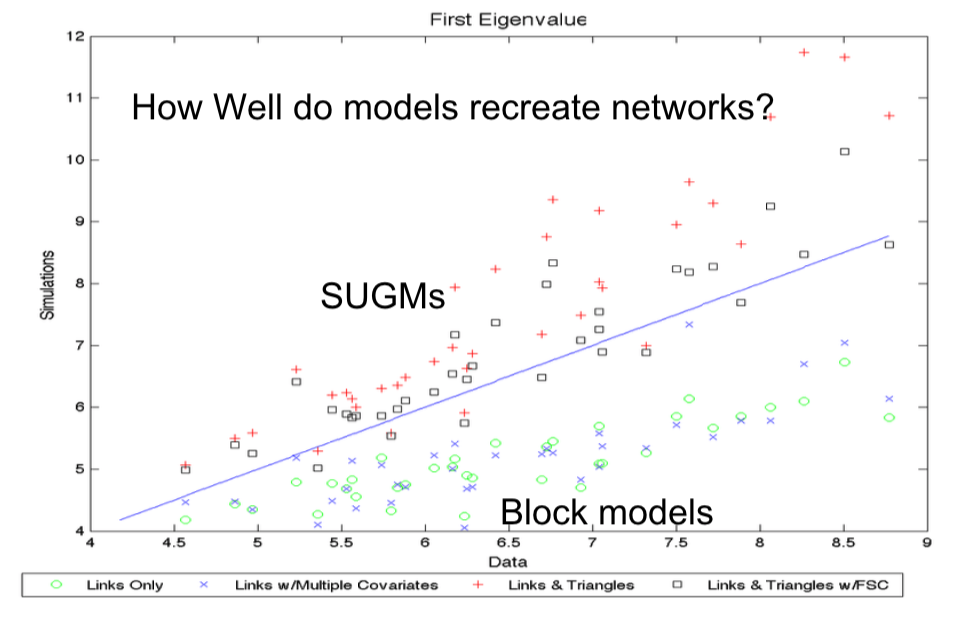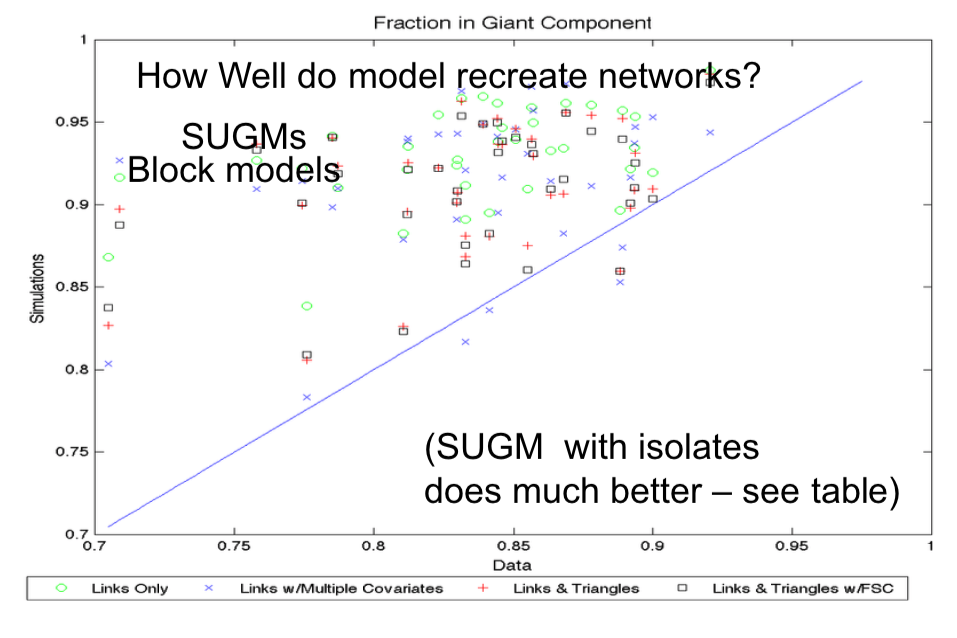## Dependencies¶

Dependencies are really important to pick up in social networks. Why? well that's the whole nature of social

• "Social" by definition generates dependencies
• Need tractable models to capture / test these
• ERGMs are rich family, but not always accurately estimable
• SERGMs and SUGMs offer easy and consistent estimation.

## Network Models¶

• Statistical models offer a medium, but also need models in context
• Understand dependencies? Friends of friends, social enforcement...
• What should we be testing for?
• Example, see lecture 4.9

## Strengths Random Networks:¶

• Generate large networks with well identified properties
• Mimic real networks (at least in some characteristics)
• Tie specific properties to specify processes

## Weaknesses of Random Network Models¶

• Missing the "Why"
• Why this process?(lattice, preferential attach...)
• Missing implications of networks structure: context or relevance
• welfare, efficiency?
• Literature is missing careful empirical analysis of many "stylized facts" (small worlds, power laws, clustering...)
• ERGMs have filling that niche, but need estimable models
• New models are emerging